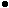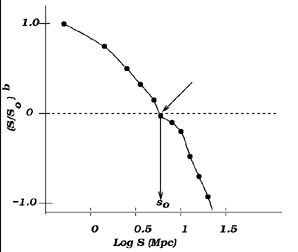3.5.5. Correlation Scales

The identification of preferred scales in the galaxy distribution provides important constraints on structure formation theories. While the identification of physical scales is best determined by measuring the power spectrum of the galaxy distribution (see Chapter 5), much information can also be learned by using a "counts-in-cells" technique. In this technique, the sky is broken up into cells of fixed volume and the number of galaxies in each 3D cell is counted. The sizes of the cells are varied and the counts are done again until some kind of convergence or pattern is seen. The analysis of the galaxy distribution as done with this counts-in-cells technique as applied to the CFA redshift survey has yielded the following scales of interest. These spatial scales serve as important constraints that structure formation theories must meet (see Chapter 5).75-80% of the survey volume is devoid of bright galaxies. Thus either these voids are preferentially inhabited by faint galaxies or indeed, the galaxies themselves, of all types, are concentrated in the intersecting surfaces of voids.The luminosity function (LF - more fully described in Chapter 6) of galaxies is fit by the parameters MB* = -19.2 ± 0.1 and= -1.1 ± 0.1. To first order, galaxy redshifts are indicative of distance which allows conversion of apparent flux into an intrinsic luminosity. While clustering (see below) introduces a second order correction to the relation between observed redshift and distance, its a minor perturbation in the determination of the LF. An accurate determination of the LF is another important quantitative result that can constrain theoretical models of structure formation. However, as we shall see in Chapter 6, the faint end slope of the LF can not be well-determined due to surface brightness bias in existing redshift surveys and furthermore is significantly steeper than the value of= -1.1 ± 0.1. In fact, a new analysis of the LF for the CFA redshift survey as a function of galaxy type has been presented by Marzke et al. (1995). They find that for some galaxy types, the faint end slope is considerable steeper than= -1.1.The two point correlation length of galaxies is 7.5h-1 Mpc. The data are shown in Figure 3-13. This correlation length is defined by the probability (in excess of random) of finding a galaxy at a distance, r, from a given other galaxy. The radius at which this probability equals unity is identified as the correlation length. A determination of the correlation length is a good descriptor of small scale clustering.Figure 3-13: The two point correlation function for groups of galaxies. The spatial correlation function is well approximated by the form (s / s0)b, where the correlation length so and the slope of the power law, b, are the fitting parameters. The dashed line indicates normalization of the power law fit which defines the correlation length. The spatial scale over which the data cross this line defines so. The Y-axis plots the logarithim of (s/so)b. The fit to a power law for this data gives a slope of -1.3 and a correlation length of 8 -1 Mpc. Adapted from Ramella et al. (1989).The cluster-cluster correlation length is20 ± 5 h-1 Mpc (see Postman et al. 1992). This is shown in Figure 3-14. The relatively large uncertainty in this length is a reflection of selection effects in making clusters catalogs; different samples of clusters give somewhat different values for the correlation length.Figure 3-14: Cluster-cluster correlation function for an X-ray flux limited sample of galaxies from Bahcall and Cen (1992). The x-axis is the spatial scale, s, in log h-1 Mpc and the Y-axis plots the logarithim of (s/so)b. Although the data set is noisy and the sample size is small, the data are consistent with a correlation length of 21 h-1 Mpc. This is equivalent to the correlation length found in numerical simulation of low density universes (see Bahcall and Cen 1992; Mo et al. 1996).